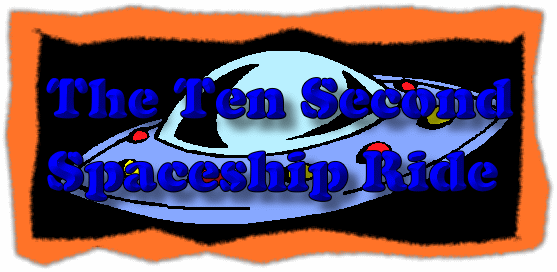# Ten Second Spaceship RideConstant acceleration, two dimensional motion demonstration

See notes below.

#### Flash

This is Zona Land Education's Ten Second Spaceship Ride. Every ten seconds the motion of the spaceship recycles. You can change the initial parameters for the motion and then see how these changes effect the path of the spaceship. Working with several examples can help you understand the relationship between position, velocity, and acceleration.

The sliders at the bottom let you adjust the motion's original conditions. Under each slider is the name of its quantity and the current value for that quantity. These quantities are:

• xo - The original position in the x-direction.
• yo - The original position in the y-direction.
• vxo - The original velocity in the x-direction.
• vyo - The original velocity in the y -direction.
• ax - The constant acceleration in the x-direction.
• ay - The constant acceleration in the y-direction.

Moving any one of the sliders will restart the motion using the new value from that slider. Once you set up a motion and no longer adjust the sliders, the motion will repeat itself about every ten seconds.

The current x- and y-positions and x- and y-velocities are shown at the top of the graph. These values will usually change as the spaceship moves. Note that the x- and y-accelerations do not change during the motion. This demonstrations shows motions with constant accelerations.

To get your thinking started, move the vxo slider over to about 1.0. You should see the spaceship moving down the x-axis.

Next, you might adjust the starting position for the motion using the xo and yo sliders. Give the spaceship a small y-velocity with the vyo slider, and you would then be moving it in two dimensions. Adjust the x- and y-accelerations with the ax and ay sliders respectively, and you will see the corresponding velocities change during the motion.

Custom Search